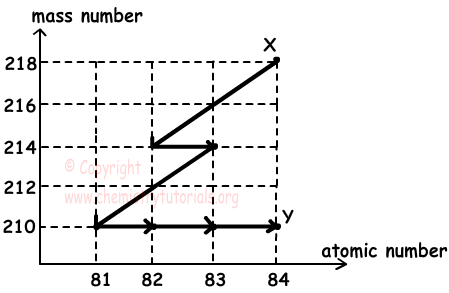EN | ES | DE | NL | RU

## Natural Nuclear Reactions and Radioactive Decays

Natural Nuclear Reactions and Radioactive Decays

In radioactive reactions, charge and mas number are conserved. Now we explain radioactive decays, radiation one by one.

Alpha (α) particles can be called Helium-4 nuclei (24He+2). After alpha decay, atomic number of nucleus decreases by 2 and mass number decreases by 4 and number of neutrons also decreases by 2.

Example:

92238U → 90234Th + 24He

86222Rn → 84218Po + 24He

Properties of α particles:

• Since they are positively charged, their ionization capability is high.
• They are affected by electric field and deviate towards negatively charged plate.
• A piece of paper can stop motion of α particles.

Beta radiation is formed during conversion of one neutron to one proton. Particle produced after this process is electron. We show it in nuclear reactions with Greek letter "β-"

01n → 11p + -10e(β-)

After beta decay, number of proton increases by one and number of neutrons decreases by one. Thus, mass number stays constant.

Example:

55137Cs → 56137Ba + -10e

13H → 23He + -10e

614C → 714N + --10e

Properties of beta particles:

• Beta particles moves with a velocity that is closer to speed of light.
• Since their charge is smaller than alpha particles, their ionization capability is lower than alpha particles.
• Since they are charged particles, they deviate in electric and magnetic fields.
• Their penetrating capability is higher than alpha particles, they can penetrate aluminum having thickness 2-3 mm.

It is also called, beta positive decay. It is denoted by +10e or β+. Positron decay is conversion of one proton to one neutron.

11p → 01n + +10e

In positron decay mass number is conserved, however, number of protons decreases by one and number of neutrons increases by one.

Example:

1938K → 1838Ar + +10e

53122I → 52122Te + +10e

2754Co → 2654Fe + +10e

Properties of positron particles:

• Since they are charged particles, they deviate in electric and magnetic fields.
• Positron particles have same property with beta particles in capability of ionizing and penetrating.

Gamma radiations are short wave length electromagnetic waves. Gamma decays occur after other radiations to emit excess energy of nucleus to become stable. Gamma radiation is shown with "γ".

In reactions it is shown as "00γ". After gamma decay, atomic number and mass number of nucleus are conserved.

Example:

1st step: 94240Pu → [92236U] +24He

2nd step: 92236U → 92236U + 00γ

Properties of gamma rays:

• They are high energy electromagnetic waves
• Since they are neutral, they do not deviate in electric and magnetic field.
• Their penetrating ability is too high.

5. Electron Capture:

Some of nuclei capture one electron on inner shell of it. This electron convert one proton to one neutron in nucleus.

11p + -10e → 01n

After electron capture, mass number is conserved, atomic number decreases by one and number of neutrons increases by 1.

Example:

2758Co + -10e → 2658Fe

47106Ag + -10e → 46106Pd

Example:

90234X + β- → Y + γ + 2β+ + α

Find number of protons and mass number of Y in given reaction above.

Solution:

Number of protons in left side of reaction is;

90+(-1)=89

Thus, number of protons in right side of reaction must be 89.

Y+1.(0) + 2.(+1) +2 =89

Y+4=89

Y=85 number of protons

Mass number of reactants must be equal to mass numbers of products.

234+0=234 mass number of reactants

Y+ 1.(0) + 2.(0) + 4=234

Y + 4=234

Y=230 mass number of Y

85230Y

Example: X does nuclear decays and converted to Y. Graph given below shows changes in the mass number vs atomic number of X.Which ones of the following statements are true?

I. X does 2 α and 4 β- decays

II. X and Y are isotopes

III. Neutron numbers of Y is 126

Solution:

In β- decay mass number stays constant and atomic number increases by 1. In α decay mass number decreases by 4 and atomic number decreases by 2.

I. As shown in the graph, X does 2 α and 4 β- decays I is true

II. We see that in graph X and Y has same atomic number so they are isotopes. II is true

III. In graph, we see atomic number of Y is 84 and mass number is 210

210-84=126 neutrons III is true.

The Original Author: